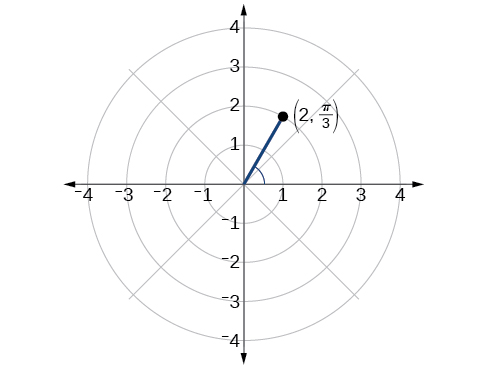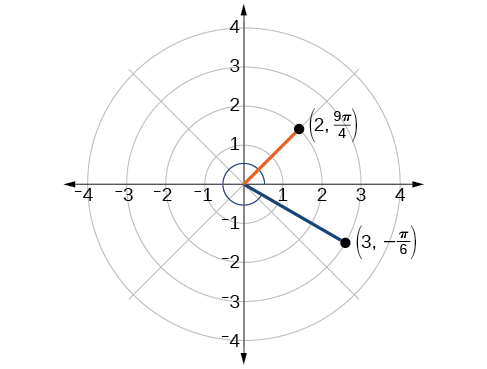10.3 Polar coordinates

 Page 1 / 8
In this section, you will:
• Plot points using polar coordinates.
• Convert from polar coordinates to rectangular coordinates.
• Convert from rectangular coordinates to polar coordinates.
• Transform equations between polar and rectangular forms.
• Identify and graph polar equations by converting to rectangular equations.

Over 12 kilometers from port, a sailboat encounters rough weather and is blown off course by a 16-knot wind (see [link] ). How can the sailor indicate his location to the Coast Guard? In this section, we will investigate a method of representing location that is different from a standard coordinate grid.

Plotting points using polar coordinates

When we think about plotting points in the plane, we usually think of rectangular coordinates $\text{\hspace{0.17em}}\left(x,y\right)\text{\hspace{0.17em}}$ in the Cartesian coordinate plane. However, there are other ways of writing a coordinate pair and other types of grid systems. In this section, we introduce to polar coordinates    , which are points labeled $\text{\hspace{0.17em}}\left(r,\theta \right)\text{\hspace{0.17em}}$ and plotted on a polar grid. The polar grid is represented as a series of concentric circles radiating out from the pole    , or the origin of the coordinate plane.

The polar grid is scaled as the unit circle with the positive x- axis now viewed as the polar axis    and the origin as the pole. The first coordinate $\text{\hspace{0.17em}}r\text{\hspace{0.17em}}$ is the radius or length of the directed line segment from the pole. The angle $\text{\hspace{0.17em}}\theta ,$ measured in radians, indicates the direction of $\text{\hspace{0.17em}}r.\text{\hspace{0.17em}}$ We move counterclockwise from the polar axis by an angle of $\text{\hspace{0.17em}}\theta ,$ and measure a directed line segment the length of $\text{\hspace{0.17em}}r\text{\hspace{0.17em}}$ in the direction of $\text{\hspace{0.17em}}\theta .\text{\hspace{0.17em}}$ Even though we measure $\text{\hspace{0.17em}}\theta \text{\hspace{0.17em}}$ first and then $\text{\hspace{0.17em}}r,$ the polar point is written with the r -coordinate first. For example, to plot the point $\text{\hspace{0.17em}}\left(2,\frac{\pi }{4}\right),$ we would move $\text{\hspace{0.17em}}\frac{\pi }{4}\text{\hspace{0.17em}}$ units in the counterclockwise direction and then a length of 2 from the pole. This point is plotted on the grid in [link] .

Plotting a point on the polar grid

Plot the point $\text{\hspace{0.17em}}\left(3,\frac{\pi }{2}\right)\text{\hspace{0.17em}}$ on the polar grid.

The angle $\text{\hspace{0.17em}}\frac{\pi }{2}\text{\hspace{0.17em}}$ is found by sweeping in a counterclockwise direction 90° from the polar axis. The point is located at a length of 3 units from the pole in the $\text{\hspace{0.17em}}\frac{\pi }{2}\text{\hspace{0.17em}}$ direction, as shown in [link] .

Plot the point $\text{\hspace{0.17em}}\left(2,\text{\hspace{0.17em}}\frac{\pi }{3}\right)\text{\hspace{0.17em}}$ in the polar grid .Plotting a point in the polar coordinate system with a negative component

Plot the point $\text{\hspace{0.17em}}\left(-2,\text{\hspace{0.17em}}\frac{\pi }{6}\right)\text{\hspace{0.17em}}$ on the polar grid.

We know that $\text{\hspace{0.17em}}\frac{\pi }{6}\text{\hspace{0.17em}}$ is located in the first quadrant. However, $\text{\hspace{0.17em}}r=-2.\text{\hspace{0.17em}}$ We can approach plotting a point with a negative $\text{\hspace{0.17em}}r\text{\hspace{0.17em}}$ in two ways:

1. Plot the point $\text{\hspace{0.17em}}\left(2,\frac{\pi }{6}\right)\text{\hspace{0.17em}}$ by moving $\text{\hspace{0.17em}}\frac{\pi }{6}\text{\hspace{0.17em}}$ in the counterclockwise direction and extending a directed line segment 2 units into the first quadrant. Then retrace the directed line segment back through the pole, and continue 2 units into the third quadrant;
2. Move $\text{\hspace{0.17em}}\frac{\pi }{6}\text{\hspace{0.17em}}$ in the counterclockwise direction, and draw the directed line segment from the pole 2 units in the negative direction, into the third quadrant.

See [link] (a). Compare this to the graph of the polar coordinate $\text{\hspace{0.17em}}\left(2,\frac{\pi }{6}\right)\text{\hspace{0.17em}}$ shown in [link] (b).

Plot the points $\text{\hspace{0.17em}}\left(3,-\frac{\pi }{6}\right)$ and $\text{\hspace{0.17em}}\left(2,\frac{9\pi }{4}\right)\text{\hspace{0.17em}}$ on the same polar grid.Converting from polar coordinates to rectangular coordinates

When given a set of polar coordinates    , we may need to convert them to rectangular coordinates . To do so, we can recall the relationships that exist among the variables $\text{\hspace{0.17em}}x,\text{\hspace{0.17em}}y,\text{\hspace{0.17em}}r,\text{\hspace{0.17em}}$ and $\text{\hspace{0.17em}}\theta .$

The sequence is {1,-1,1-1.....} has
how can we solve this problem
Sin(A+B) = sinBcosA+cosBsinA
Prove it
Eseka
Eseka
hi
Joel
June needs 45 gallons of punch. 2 different coolers. Bigger cooler is 5 times as large as smaller cooler. How many gallons in each cooler?
7.5 and 37.5
Nando
find the sum of 28th term of the AP 3+10+17+---------
I think you should say "28 terms" instead of "28th term"
Vedant
the 28th term is 175
Nando
192
Kenneth
if sequence sn is a such that sn>0 for all n and lim sn=0than prove that lim (s1 s2............ sn) ke hole power n =n
write down the polynomial function with root 1/3,2,-3 with solution
if A and B are subspaces of V prove that (A+B)/B=A/(A-B)
write down the value of each of the following in surd form a)cos(-65°) b)sin(-180°)c)tan(225°)d)tan(135°)
Prove that (sinA/1-cosA - 1-cosA/sinA) (cosA/1-sinA - 1-sinA/cosA) = 4
what is the answer to dividing negative index
In a triangle ABC prove that. (b+c)cosA+(c+a)cosB+(a+b)cisC=a+b+c.
give me the waec 2019 questions
the polar co-ordinate of the point (-1, -1)ByByBy